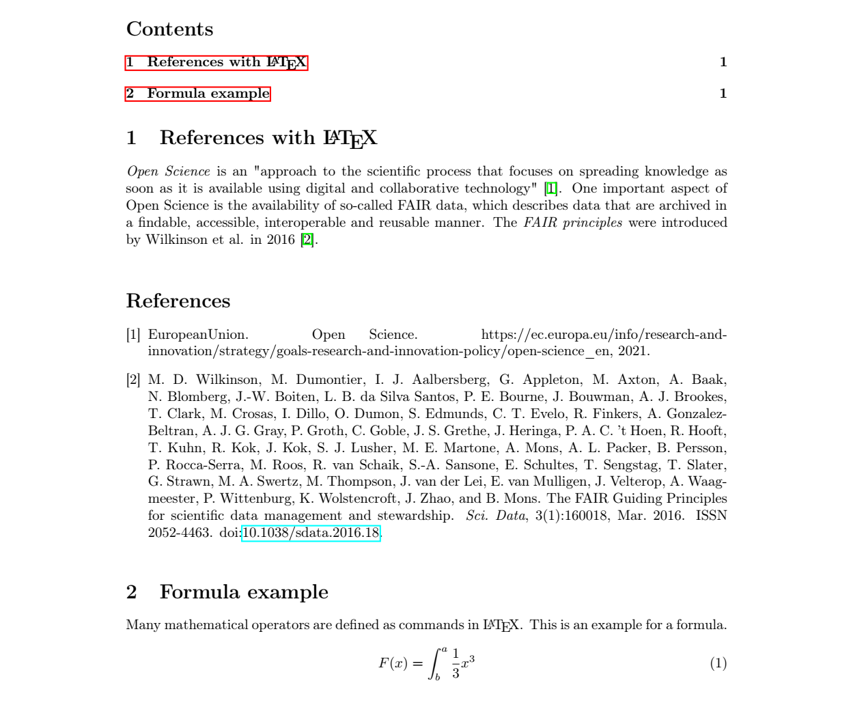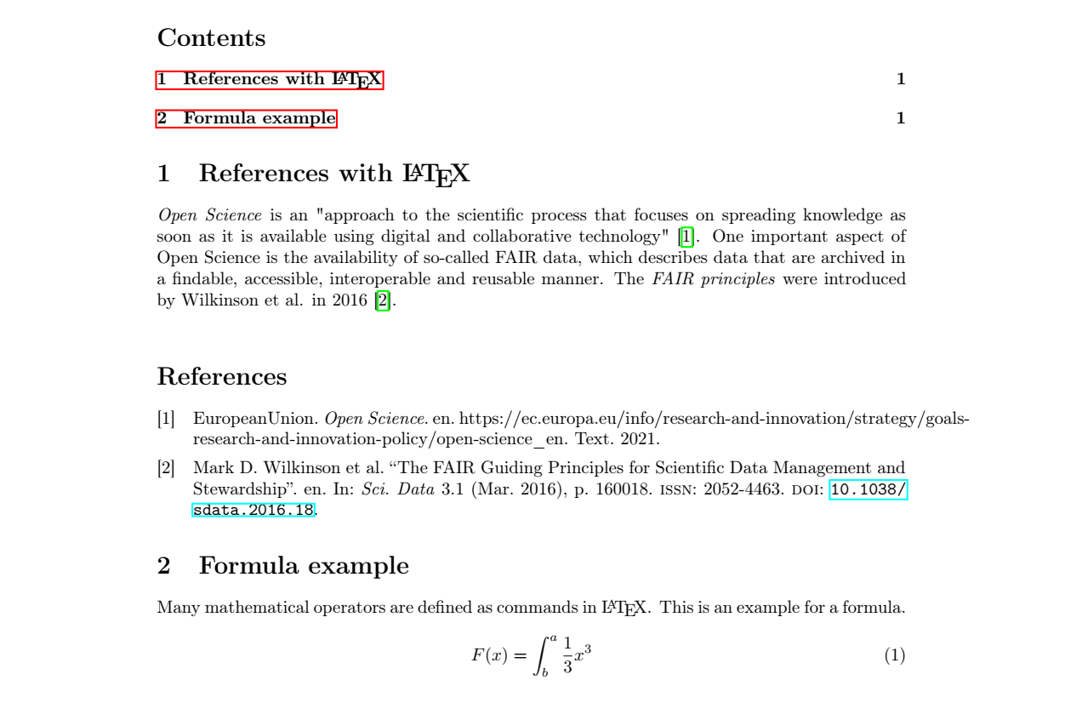Reference Management

# Reference Management in LaTeX

BibTeX

LaTeX is a typesetting system for document production that is commonly used for scientific texts such as articles, theses, and books. It is particularly useful for writing of mathematical formulas, something that is complicated with visual programs such as MS Word, and structured texts as the table of content is generated and updated automatically. LaTeX is based on the principle that authors should solely have to take care of the content and not have to deal with the document formatting. The result are documents with a professionally looking typography without spending any time on it. Although it takes a bit of time to get started with LaTeX, the invested time pays off later, for example when an article can be transferred effortless from one journal-template to another.
Text representation and format are separated in LaTeX. Each document consists of a preamble which contains all formatting information (added as packages with \usepackage{}), followed by the actual content which is framed by \begin{document} and \end{document}.

LaTeX can be installed locally or one can use the online LaTeX editor Overleaf which is excellent for collaborative writing.

LaTeX comes with its own integrated reference management system: BibTeX. The desired reference style is set in the preamble while the reference information itself is attached as a structured text file (BibTeX file, .bib). Each reference has a cite key (commonly author & year) that is referred to in the text.
It is possible to generate .bib files manually (several publishers allow to export citations as BibTex), however, it is much more convenient to use reference management software that can generate .bib files:

More flexible citation styles can be generated with the natbib package, a re-implementation of BibTeX, or the biblatex package.
You can find more useful information in the LaTeX-course from the University of Stavanger, Bib(La)TeX-guide from the University of Oslo as well as on many websites, including the LaTeX wikibook resource and Overleaf.

A template for LaTeX PhD theses at the University of Bergen can be found in the thesis portal (Avhandlingsportalen).
There is also several example documents from UiB on Overleaf.

Do not hesitate to contact us if you need help or are interested in a course.

## Example for chronological references with natbib

%preamble
%%%
%format
\documentclass{article}
\usepackage{a4wide}
%encoding & font
\usepackage[utf8x]{inputenc}
\usepackage[T1]{fontenc}
%spellcheck
\usepackage[english]{babel}
%references with natbib
\usepackage[square,numbers]{natbib}
\bibliographystyle{abbrvnat}
\usepackage{doi}

%mathematics
\usepackage{mathtools}

\begin{document}

\tableofcontents

\section{References with \LaTeX}

\textit{Open Science} is an "approach to the scientific process that focuses on spreading knowledge as soon as it is available using digital and collaborative technology" \cite{EuropeanUnion2021}. One important aspect of Open Science is the availability of so-called FAIR data, which describes data that are archived in a findable, accessible, interoperable and reusable manner. The \textit{FAIR principles} were introduced by Wilkinson et al. in 2016 \cite{Wilkinson2016}.

\bibliography{my_library}

\section{Formula example}

Many mathematical operators are defined as commands in \LaTeX. This is an example for a formula.
\begin{equation}
F(x) = \int^a_b \frac{1}{3}x^3
\end{equation}

#### Compiled documentPhoto:
Jenny Ostrop, UiB

## Example for chronological references with biblatex

%preamble
%%%
%format
\documentclass{article}
\usepackage{a4wide}
%encoding & font
\usepackage[utf8x]{inputenc}
\usepackage[T1]{fontenc}
%spellcheck
\usepackage[english]{babel}
%references with biblatex
\usepackage[backend=biber,style=numeric,bibstyle=numeric,sorting=none]{biblatex}
\usepackage{doi}

%mathematics
\usepackage{mathtools}

\begin{document}

\tableofcontents

\section{References with \LaTeX}

\textit{Open Science} is an "approach to the scientific process that focuses on spreading knowledge as soon as it is available using digital and collaborative technology" \cite{EuropeanUnion2021}. One important aspect of Open Science is the availability of so-called FAIR data, which describes data that are archived in a findable, accessible, interoperable and reusable manner. The \textit{FAIR principles} were introduced by Wilkinson et al. in 2016 \cite{Wilkinson2016}.

\printbibliography

\section{Formula example}

Many mathematical operators are defined as commands in \LaTeX. This is an example for a formula.
\begin{equation}
F(x) = \int^a_b \frac{1}{3}x^3
\end{equation}

\end{document}

#### Compiled document